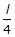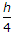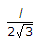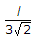# Civil Engineering - Theory of Structures

Exercise : Theory of Structures - Section 2
1.
By applying the static equations i.e. ΣH = 0, ΣV = 0 and ΣM = 0, to a determinate structure, we may determine
supporting reactions only
shear forces only
bending moments only
internal forces only
all the above.
Explanation:
No answer description is available. Let's discuss.

2.
The general expression for the B.M. of a beam of length l is the beam carries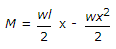a uniformly distributed load w/unit length
a load varying linearly from zero at one end to w at the other end
an isolated load at mid span
none of these.
Explanation:
No answer description is available. Let's discuss.

3.
The ratio of the length and diameter of a simply supported uniform circular beam which experiences maximum bending stress equal to tensile stress due to same load at its mid span, is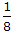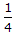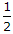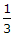1.0
Explanation:
No answer description is available. Let's discuss.

4.
If a solid shaft (diameter 20 cm, length 400 cm, N = 0.8 x 105 N/mm2) when subjected to a twisting moment, produces maximum shear stress of 50 N/mm2, the angle of twist in radians, is
0.001
0.002
0.0025
0.003
0.005